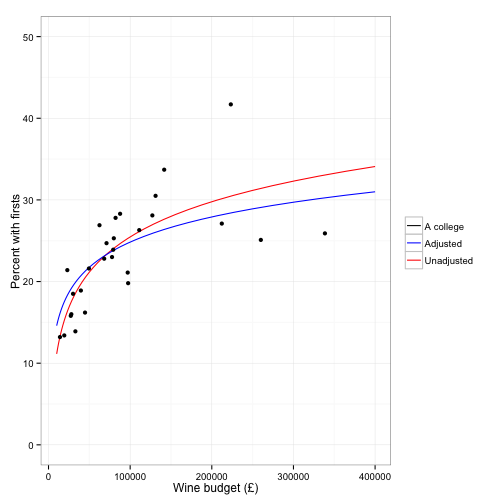# Linear regression with R

Regression with R

# Regression with R

This is an R Markdown analysis log. This document was compiled at: 2014-02-11 13:41:05

First we need to load the packages I'll be using.

require(RCurl)  # this is due to an issue with https, R and github

## Loading required package: RCurl

require(MASS)  # for robust regression

## Loading required package: MASS

require(car)  # AVP plots

## Loading required package: car

require(reshape)  # During the last plot I reshape some data

## Loading required package: reshape
##
## Attaching package: 'reshape'
##
## The following objects are masked from 'package:plyr':
##
##     rename, round_any

require(ggplot2)  # last plot is a ggplot

## Loading required package: ggplot2

options(scipen = 99)  # no scientific notation

location_data <- "location of data"
location_output <- "output folder"


I pulled this data from a variety of sources. As this isn't a serious analysis, I won't bother citing.

So that others won't have to crawl through different websites, I have saved the data into a gist. So first thing I need to do is load it up.

data <- getURL("https://gist.github.com/epijim/8819934/raw/6c76df80eb095065a9ce0fa4b8f94410ad528fed/college_data.csv")
data <- read.csv(text = data, stringsAsFactors = F)


# Check data

Look at the variable types

str(data)

## 'data.frame':    31 obs. of  37 variables:
##  $College : chr "Christ's" "Churchill" "Clare" "Clare Hall" ... ##$ Grad_coll             : logi  FALSE FALSE FALSE TRUE FALSE TRUE ...
##  $Wine_budget : int 71055 87685 79989 17400 79254 17510 77798 131127 23028 30051 ... ##$ Fellows               : int  84 165 120 67 54 68 65 87 56 118 ...
##  $Students : int 617 770 816 181 483 633 696 650 780 722 ... ##$ Grads                 : int  208 290 312 181 223 633 271 220 288 220 ...
##  $PhD : int 127 190 190 96 145 421 151 121 151 102 ... ##$ Other                 : int  81 100 122 85 78 212 120 99 137 118 ...
##  $Rating : chr "B" "B" "A" "B" ... ##$ Part_time             : chr  "Yes" "Yes" "Yes" "Yes" ...
##  $Undergrad_applications: int 451 350 573 NA 200 NA 548 503 260 261 ... ##$ Undergrad_acceptances : int  99 89 134 NA 68 NA 97 126 95 119 ...
##  $Undergrad_acceptRate : int 22 25 23 NA 34 NA 18 25 37 46 ... ##$ Founded               : int  1505 1960 1326 1965 1352 1964 1800 1584 1966 1869 ...
##  $Percent_male : int 58 71 52 47 60 54 66 51 63 53 ... ##$ Percent_female        : int  42 29 48 53 40 46 34 49 37 47 ...
##  $Fixed_assets : int 66602000 105978346 70707000 10579203 191233087 33160032 86798000 152640692 43509000 64000000 ... ##$ Tompkin_1997          : int  3 15 11 NA 23 NA 12 7 13 22 ...
##  $Tompkin_1998 : int 2 13 6 NA 18 NA 11 5 12 21 ... ##$ Tompkin_1999          : int  1 20 15 NA 8 NA 16 5 19 21 ...
##  $Tompkin_2000 : int 1 15 9 NA 10 NA 8 3 21 18 ... ##$ Tompkin_2001          : int  1 9 6 NA 20 NA 10 2 13 17 ...
##  $Tompkin_2002 : int 4 10 3 NA 18 NA 8 2 20 16 ... ##$ Tompkin_2003          : int  2 9 6 NA 7 NA 12 1 20 17 ...
##  $Tompkin_2004 : int 2 19 4 NA 10 NA 17 1 15 25 ... ##$ Tompkin_2005          : int  4 18 9 NA 16 NA 15 5 13 24 ...
##  $Tompkin_2006 : int 6 13 12 NA 8 NA 11 1 19 22 ... ##$ Tompkin_2007          : int  2 15 17 NA 8 NA 3 1 14 21 ...
##  $Tompkin_2008 : int 8 6 13 NA 9 NA 12 2 21 22 ... ##$ Tompkin_2009          : int  13 7 18 NA 10 NA 15 2 21 20 ...
##  $Tompkin_2010 : int 12 3 8 NA 13 NA 15 1 22 21 ... ##$ Tompkin_2011          : int  6 10 4 NA 12 NA 17 2 21 23 ...
##  $Tompkin_2012 : int 9 5 11 NA 3 NA 20 2 19 22 ... ##$ Tompkin_2013          : int  8 5 11 NA 16 NA 12 4 20 21 ...
##  $Tompkin_Mean09to13 : num 4.9 11.3 9.6 NA 12.3 NA 12.6 2.7 17.8 20.8 ... ##$ TompkinsScore_2013    : num  66.8 68.2 66.1 NA 64.9 ...
##  $Firsts_2013 : num 24.7 28.3 25.3 NA 23.9 NA 23 30.5 21.4 18.5 ...  # Set up the data how I want it Just in case, I'll duplicate college as a string variable, and make grad college a factor. data$College_char <- as.character(data$College) data$Grad_coll_fact <- as.factor(data$Grad_coll)  Since I'm dropping some colleges, I'll replicate data as sample, to signify it's the analysis sample DROP: Lucy Cavendish, as no wine budget, and the Grad colleges, as they aren't ranked. sample <- subset(data, College_char != "Lucy Cavendish") sample <- subset(sample, Grad_coll == FALSE)  Now general tidying - Make college name, row name. Add the age of college and members in a college. And make a log of the wine budget. sample <- data.frame(sample[, -1], row.names = sample[, 1]) sample$Age_College <- 2014 - sample$Founded sample$Members <- sample$Fellows + sample$Students
sample$log_Wine_budget <- log(sample$Wine_budget)


# Model the data!

## First by hand

R has functions to make running regressions easy. But it's good to know how they work. So first I'll do a simple analysis by hand of $$y=\alpha+\beta_1 X_1$$.

x <- sample$log_Wine_budget y <- sample$Firsts_2013
x_bar <- mean(x)
y_bar <- mean(y)
beta_hat_1 <- sum((x - x_bar) * (y - y_bar))/sum((x - x_bar)^2)
beta_hat_0 <- y_bar - beta_hat_1 * x_bar
cat(beta_hat_0, beta_hat_1)

## -46.16 6.221


So here we have the intercept, and the coefficient for log of the wine budget, in that order.

## Now using a command

simple <- lm(Firsts_2013 ~ log_Wine_budget, data = sample)
summary(simple)

##
## Call:
## lm(formula = Firsts_2013 ~ log_Wine_budget, data = sample)
##
## Residuals:
##    Min     1Q Median     3Q    Max
## -7.152 -2.136 -0.084  1.858 11.237
##
## Coefficients:
##                 Estimate Std. Error t value  Pr(>|t|)
## (Intercept)      -46.157     11.024   -4.19   0.00029 ***
## log_Wine_budget    6.221      0.986    6.31 0.0000011 ***
## ---
## Signif. codes:  0 '***' 0.001 '**' 0.01 '*' 0.05 '.' 0.1 ' ' 1
##
## Residual standard error: 4.15 on 26 degrees of freedom
## Multiple R-squared:  0.605,  Adjusted R-squared:  0.59
## F-statistic: 39.8 on 1 and 26 DF,  p-value: 0.00000111


So why did I use the log? Below is the model with raw wine budget.

raw_fit <- lm(Firsts_2013 ~ Wine_budget, data = sample)
coef(raw_fit)

## (Intercept) Wine_budget
## 18.32024204  0.00005192

summary(raw_fit)$r.squared  ##  0.3973  So the r-squared suggests this doesn't fit the data as well. So without even looking at whether the assumptions behind the regression are right, it looks like log is a lot better. Usually you look at the fit by looking at the residuals, but here it's pretty easy to see in the raw data (below) that a log curve is going to be better.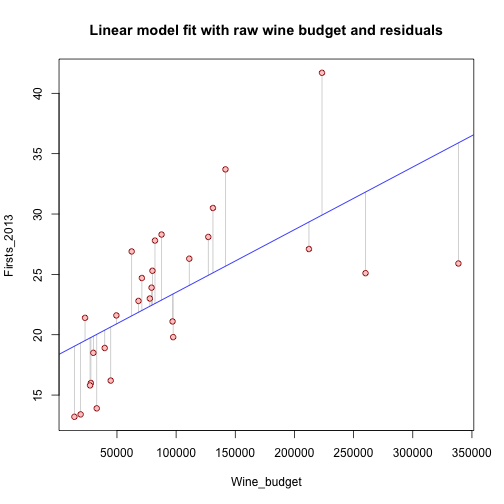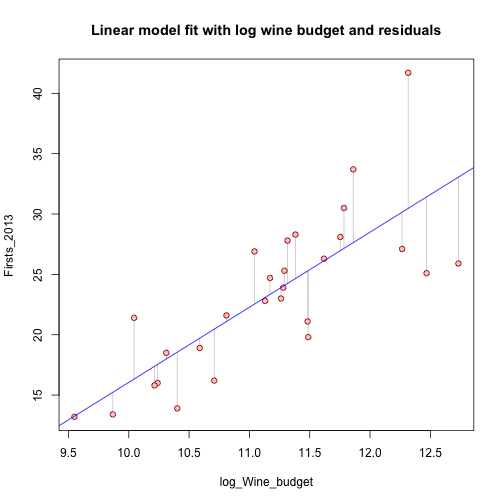Now, a model also adjusting for the number of members in a college, the age and the percent female. ols <- lm(Firsts_2013 ~ Members + log_Wine_budget + Age_College + Percent_female, data = sample) summary(ols)  ## ## Call: ## lm(formula = Firsts_2013 ~ Members + log_Wine_budget + Age_College + ## Percent_female, data = sample) ## ## Residuals: ## Min 1Q Median 3Q Max ## -6.141 -2.498 -0.524 1.757 10.180 ## ## Coefficients: ## Estimate Std. Error t value Pr(>|t|) ## (Intercept) -29.98560 15.34763 -1.95 0.0630 . ## Members 0.00479 0.00415 1.15 0.2608 ## log_Wine_budget 4.44586 1.51712 2.93 0.0075 ** ## Age_College 0.00575 0.00469 1.23 0.2319 ## Percent_female -0.05144 0.04886 -1.05 0.3034 ## --- ## Signif. codes: 0 '***' 0.001 '**' 0.01 '*' 0.05 '.' 0.1 ' ' 1 ## ## Residual standard error: 4.14 on 23 degrees of freedom ## Multiple R-squared: 0.652, Adjusted R-squared: 0.592 ## F-statistic: 10.8 on 4 and 23 DF, p-value: 0.0000449  R is smart enough to know that if you put plot, then a model results, you want to see the fit (below). plot(ols, las = 1)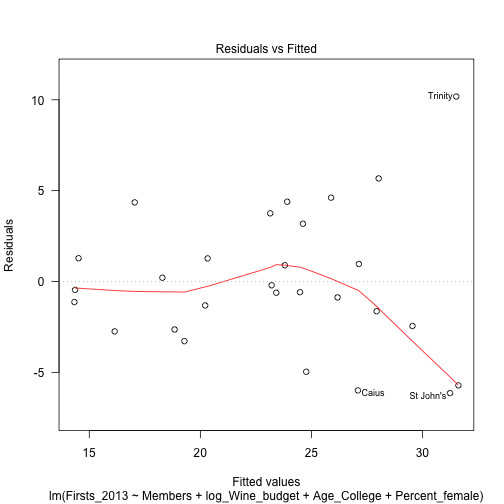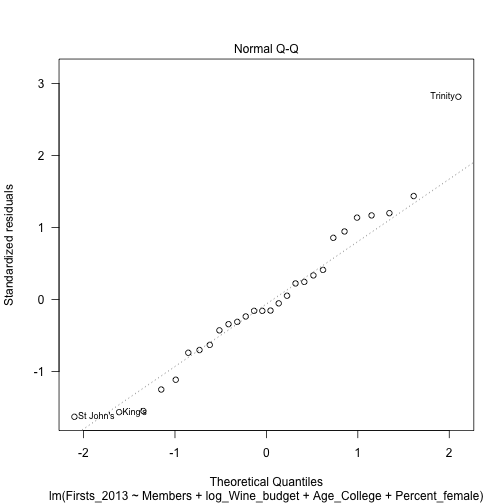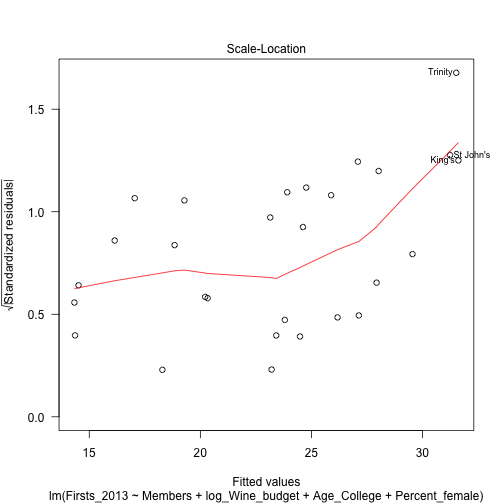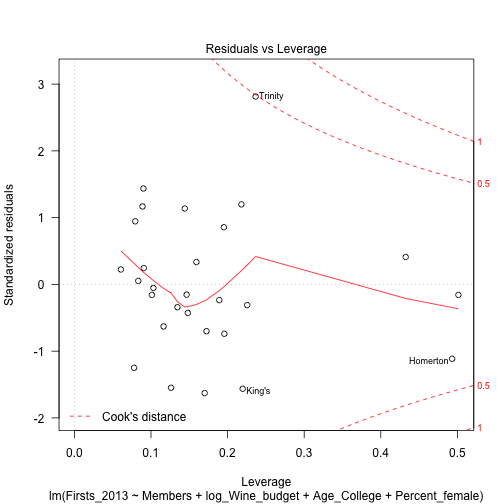# Cook's D We can display the observations that have relatively large values of data. A conventional cut-off point is 4 over n, where n is the number of observations in the data set. We will use this criterion to select the values to display. d1 <- cooks.distance(ols) r <- stdres(ols) a <- cbind(sample, d1, r) a[d1 > 4/nrow(sample), c("Firsts_2013", "Members", "Age_College", "log_Wine_budget", "Percent_female")]  ## Firsts_2013 Members Age_College log_Wine_budget Percent_female ## Homerton 16.0 1414 38 10.24 63 ## Trinity 41.7 1245 468 12.32 37  # Influence plot influencePlot(ols, id.method = "College_char", main = "Influence Plot", sub = "Circle size is proportial to Cook's Distance")## StudRes Hat CookD ## Murray Edwards -0.1543 0.5013 0.07071 ## Trinity 3.3988 0.2366 0.70059  # Compare two regressions in a plot Set the wine budget we want to plot over attach(sample) # save typing it again and again wine_min <- log(10000) wine_max <- log(400000) wine_step <- 40 plot_data <- data.frame(log_Wine_budget = seq(from = wine_min, to = wine_max, length.out = wine_step))  Simple OLS model pred_simple <- data.frame(predict(simple, plot_data, interval = "predict"))  Add in other variables needed at means plot_data$Age_College <- mean(Age_College)
plot_data$Percent_female <- mean(Percent_female) plot_data$Members <- mean(Members)


Final OLS model

ols <- lm(Firsts_2013 ~ log_Wine_budget + Age_College + Percent_female + Members,
data = sample)
pred_ols <- data.frame(predict(ols, plot_data, interval = "predict"))
detach(sample)


Merge plots and make wine budget in a readable scale.

plot_data$pred_simple <- pred_simple$fit
plot_data$pred_ols <- pred_ols$fit
plot_data$Wine_budget <- exp(plot_data$log_Wine_budget)


## Plot it

plot_data_gg <- plot_data[, c("Wine_budget", "pred_simple", "pred_ols")]
plot_data_gg <- melt(plot_data_gg, id.vars = "Wine_budget")

# colour of the legend
cols <- c(#000099 = "black", pred_simple = "red", pred_ols = "blue")

ggplot(plot_data_gg, aes(x = Wine_budget, y = value, colour = variable), xlab = "test",
) + geom_line() + ylim(0, 50) + geom_point(data = sample, aes(x = Wine_budget,
y = Firsts_2013, colour = "#000099"), show_guide = FALSE) + coord_fixed(ratio = 10000/1) +
theme_bw() + xlab("Wine budget (£)") + ylab("Percent with firsts") + theme(legend.title = element_blank()) +
scale_colour_manual(values = cols, breaks = c("#000099", "pred_ols", "pred_simple"),